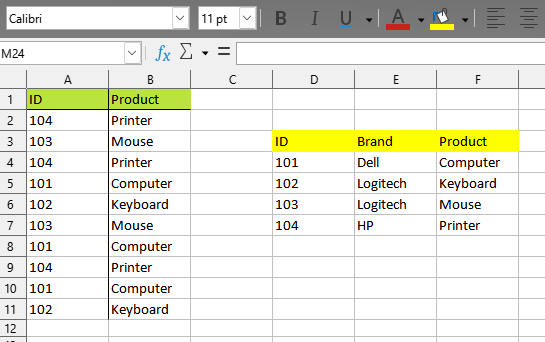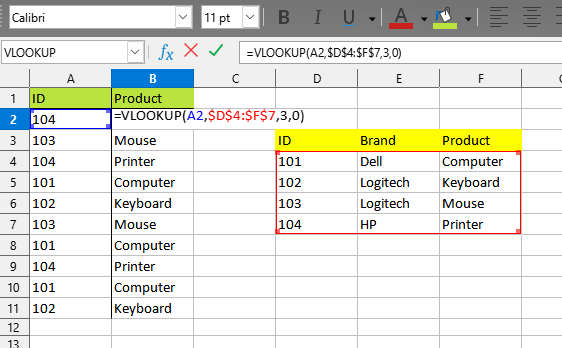# How to write LibreOffice Calc -StarBasic Macro Code For " Vlookup "? Which I get Easily in Formula and Functions in LO-Calc

HI Friends,

How to write code for " Vlookup " Functions in LibreOffice - StarBasic Macro To achieve results…
Here, the Screen Shots…Sorry for NOT providing you with Star-Basic

``````def dict_lookup(*_):
doc = XSCRIPTCONTEXT.getDocument()
sheet = doc.Sheets["Tabelle2"]
referenz= sheet["D4:F7"].DataArray
referenz = {ID:(brand, product) for ID, brand, product in referenz}

target_range = sheet["A2:B11"]
data = target_range.DataArray
out = [(ID, referenz[ID]) for ID, _ in data]
target_range.DataArray = out
``````

I’m not sure I understand your problem correctly. Are you asking how to fill in the values in column B? Or how to write the VLOOKUP() function with the correct parameters in this column? Or how to pass parameters to a user-defined function and get the result?

Hi JohnSun,

@sv.thiyagarajan Oh, it will be a lot of text, a lot. You’ll have to wait while I write it all down

The first two options for solving the problem - filling a column - can only be performed by a procedure, a subroutine (a function cannot change cells on a sheet, it only returns values).

Let’s start with the second case - we will enter the formula
`=VLOOKUP(<left cell in current row>, <fixed range to search>, 2, 0)`
in all cells of column B until the end of the data table. By the way, in your sample data, the ID column in the lookup table is sorted in ascending order. This means that you could specify 1 instead of 0 for the last parameter in the function - this makes the function much faster.

The most difficult part of the task is to determine how many cells are to be filled. VBA has a `UsedRange` that more or less accurately determines the range of cells used (often “less”, but this is not our problem, but Excel users). There is no such thing here. Therefore, users have long come up with many different ways to find the last filled cell.

This is one of many ways. Since we are looking for the last filled cell in column A, we first get the entire column (as a range), and then access this range with the query “What cells do you have empty?”. There is a special method for this, which is called - .queryEmptyCells

As a result, we will get either one range (if column A is completely empty, it will be something like \$A\$1:\$A\$1048576), or several ranges, if there are empty cells between the filled cells in column A, or not a single range - the entire column A it’s filled (getCount will return this number to us). Now it’s simple: if 0, then fill the entire column B with the formula, otherwise look where the last (or only) range of empty cells begins and subtract 1 from the row number.

Frankly, this is not a very accurate way. If the column is filled completely, but at the beginning or in the middle there will be one or more empty cells, then the row number will be determined incorrectly. But such a situation occurs so rarely that it is not necessary to complicate the macro code with additional checks - if at some point the macro does not work correctly, you will only need to correct the data in the sheet and execute the script again.

Now you know the first and last cell in the range where the formula should be. Here you have several possibilities.
You can put the formula in cell B2 and use the fillAuto(com.sun.star.sheet.FillDirection.TO_BOTTOM, 1) method on the desired range. It’s like manually entering one formula and using CTRL+D to fill in the rest of the cells.

Or you can enter one array formula in the whole range at once - .setArrayFormula(“VLOOKUP(A2:A…;\$D\$4:\$F\$7;3;1)”)

This is how the second problem is solved - you filled column B with a formula.

To complete the first task, do the same, then have Calc calculate the values for the formulas you just inserted (this is optional, but recommended). And now a very simple trick: read the computed values and write them back - .setDataArray(.getDataArray())

``````Sub fillWithVLOOKUP()
Dim oSheet As Variant
Dim oColumn As Variant
Dim nCount As Long
Dim oEmptyCells As Variant
Dim nLastRow As Long
Dim oTargetRange As Variant
oSheet = ThisComponent.getCurrentController().getActiveSheet()
oColumn = oSheet.getColumns().getByIndex(0)
oEmptyCells = oColumn.queryEmptyCells()

nCount = oEmptyCells.getCount()
If nCount = 0 Then
nLastRow = oSheet.getRows().getCount() - 1
Else
If nLastRow < 1 Then Exit Sub
EndIf
oTargetRange = oSheet.getCellRangeByPosition(1, 1, 1, nLastRow)

'	oTargetRange.getCellByPosition(0, 0).setFormula("=VLOOKUP(A2;\$D\$4:\$F\$7;3;0)") '
'	oTargetRange.fillAuto(com.sun.star.sheet.FillDirection.TO_BOTTOM, 1) '
' OR '
oTargetRange.setArrayFormula("VLOOKUP(A2:A" & (nLastRow+1) & ";\$D\$4:\$F\$7;3;1)")

Rem Replace formulas with values
ThisComponent.calculate()
oTargetRange.setDataArray(oTargetRange.getDataArray())
End Sub
``````

And a user-defined function is very simple, almost the same as in VBA. The only difference is that when you pass \$D\$4:\$F\$7 to the function via the parameter, then in the VBA inside the function you get a range, and here you get an array of values from the specified cells.
That is, it is enough to describe the header of the function

`Function my_VLOOKUP(what As Variant, where As Variant, nColumn As Long) As Variant`

(the fourth parameter won’t be needed, you won’t process it anyway - at least for now)

Now make sure you get the correct parameters. For example, that the specified column number is not less than the first and not more than the last available. You can check if the array is passed in the second parameter, if the value to look for in the first parameter is not empty, and so on.
And now just loop through all the cells in the first column of the array. As soon as the value in this cell matches the one you are looking for, return the result and complete the function:

``````Function my_VLOOKUP(what As Variant, where As Variant, nColumn As Long) As Variant
Dim i As Long
If nColumn < LBound(where,2) Then Exit Function
If nColumn > UBound(where,2) Then Exit Function
For i = LBound(where,1) To UBound(where,1)
If where(i, 1) = what Then
my_VLOOKUP = where(i,nColumn)
Exit Function
EndIf
Next i
End Function
``````